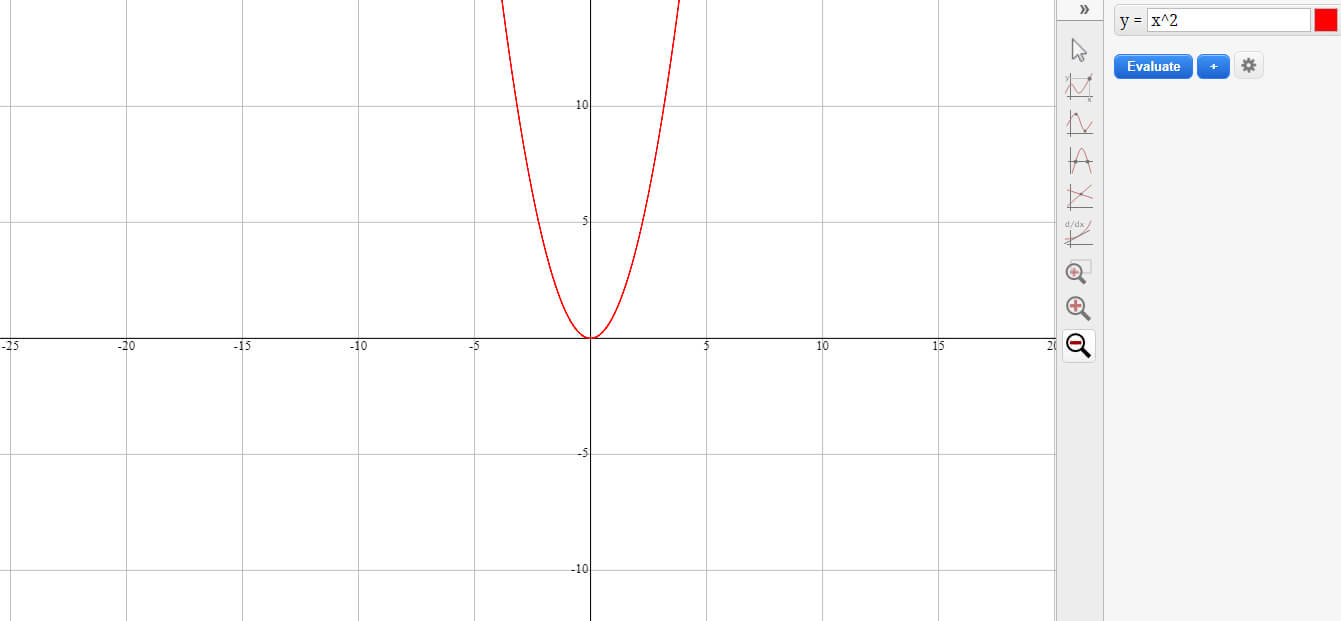# graphing calculator and multiple function plottergraphing calculator and multiple function plotter, with support for standard algebraic functions in the Cartesian plane. Features include fast, accurate graphing and full mouse zoom and pan support. Additionally, there are many graphing tools available, such as tracing a function, finding a root, finding the derivative, etc..

Remember, your mobile device has limited memory and processing power. Be kind to it! (Keep your function simple...)

## Some graphs to try

The grapher will accept any of the following functions (use the notation shown):

• Straight lines: (like 3x - 2)
• Polynomials: (like x^3 + 3x^2 - 5x + 2)
• Any of the trigonometric functions: sin(x), cos(x/2), tan(2x), csc(3x), sec(x/4), cot(x)
• The inverse trigonometric functions: arcsin(x), arccos(x), arctan(x), arccsc(x), arcsec(x), arccot(x)
• Exponential (e^x) and logarithm (ln(x) for natural log and log(x) for log base 10)
• Absolute value: use "abs" like this: abs(x)
• The hyperbolic functions and their inverses: sinh(x), cosh(x), tanh(x), arcsinh(x), arccosh(x), arctanh(x)
• Sign (1 if the sign is positive, ?1 if the sign of the function is negative). For example, try sign(sin(x))

In fact, you can use most of the javascript math functions, including

• ceiling: ceil(x) and round: round(x)
• square root: sqrt(x)

You can also use any combinations of the above, like "ln(abs(x)".

## How to draw a graph from a function?

If the table of values ​​is provided, simply place at least two pairs of points on a graph and to connect them by a straight line. However, if only the equation of the function is known, then one must first create a table of values. We can plot the graph of an affine function using the parameters a and b of the function. Indeed, if we know the intercept (the parameter b) and the rate of change (the parameter a), we can easily draw the line in a Cartesian plane. Let's see the technique by plotting the following equation using parameters a and b: y = 2x − 3. In this equation, parameter a is equal to 2 and parameter b is equal to -3. We can therefore say that the rate of change is 2 and that the intercept is -3. The parameter b represents the intercept (the value of y when x = 0). This will give us the first point to place in the graph, the point (0,−3).